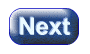created 08/26/97; revised 08/12/00; 07/22/03

# CHAPTER 6 — Scaling and Unit Vectors

This chapter discusses how to multiply a vector by a real number (called scaling), and how this is used to construct unit vectors. Unit vectors are used to show direction in 3D space, and are essential for 3D graphics.

• Scaling.
• Unit vectors.
• Normalizing a vector.
• Variables in vector equations.

### QUESTION 1:Change the elements of this column matrix: (3, 4)T so that the vector it represents is twice as long and remains pointing in the same direction. (Officially you don't know how to do this. Take a guess.)